Calculus 2 : Parametric, Polar, and Vector

Example Questions

1 2 54 55 56 57 58 59 60 62 Next →

Example Question #106 : Vector Calculations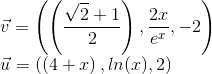Calculate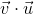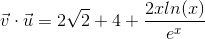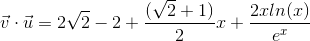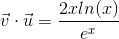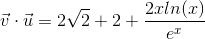Explanation:

Calculate the dot product of the 2 vectors.

In general,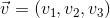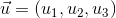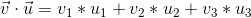Solution: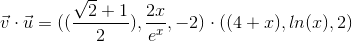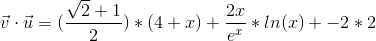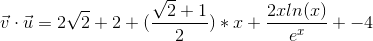Example Question #107 : Vector Calculations

Find the sum of the vectors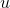and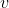if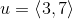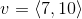.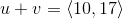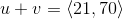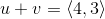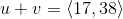Explanation:

For the two vectors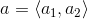and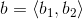The sum of the two vectors a+b is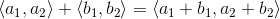For the vectors u and v in this problem the sum u+v is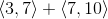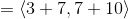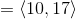1 2 54 55 56 57 58 59 60 62 Next →